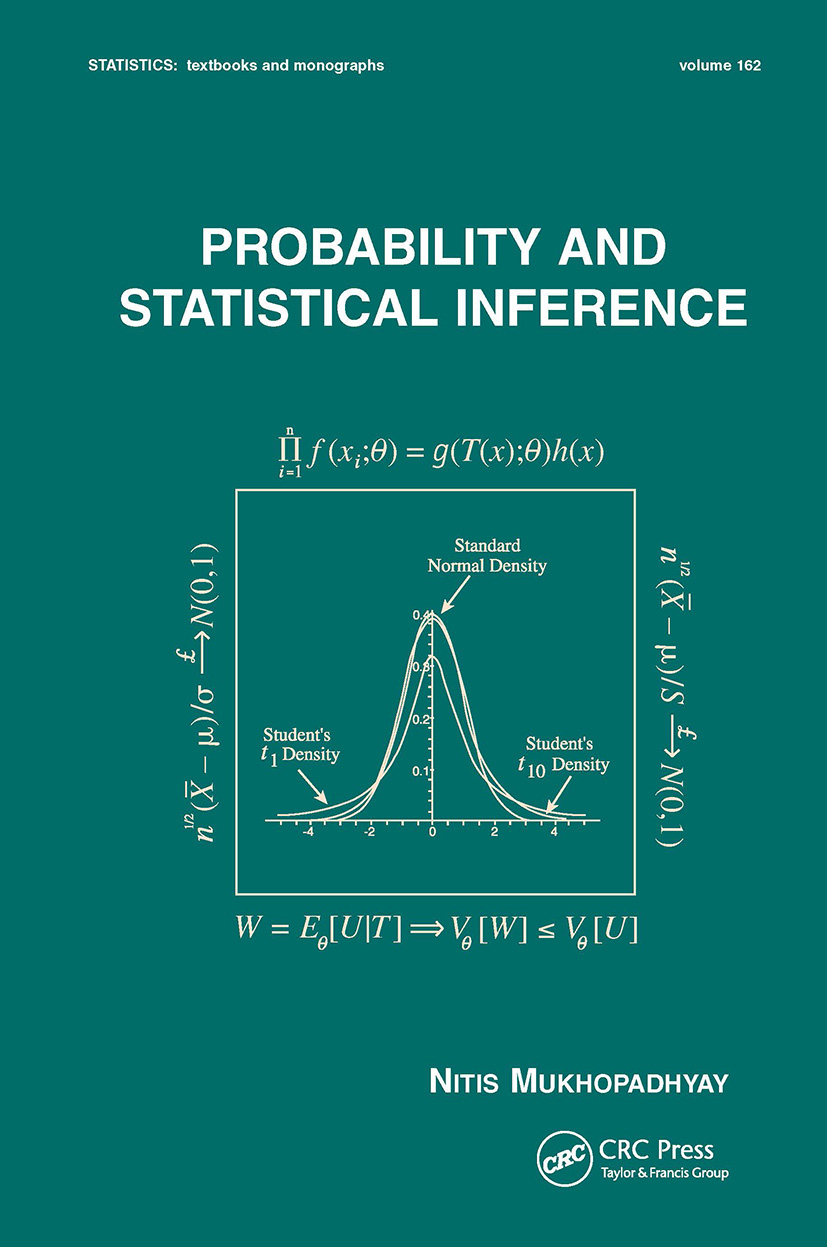Probability and Statistical Inference

1st Edition

CRC Press

665 pages

Purchasing Options:\$ = USD
Hardback: 9780824703790
pub: 2000-03-22
SAVE ~\$28.00
\$140.00
\$112.00
x

FREE Standard Shipping!

Description

Priced very competitively compared with other textbooks at this level!

This gracefully organized textbook reveals the rigorous theory of probability and statistical inference in the style of a tutorial, using worked examples, exercises, numerous figures and tables, and computer simulations to develop and illustrate concepts.

Beginning with an introduction to the basic ideas and techniques in probability theory and progressing to more rigorous topics, Probability and Statistical Inference

• studies the Helmert transformation for normal distributions and the waiting time between failures for exponential distributions

• develops notions of convergence in probability and distribution

• spotlights the central limit theorem (CLT) for the sample variance

• introduces sampling distributions and the Cornish-Fisher expansions

• concentrates on the fundamentals of sufficiency, information, completeness, and ancillarity

• explains Basu's Theorem as well as location, scale, and location-scale families of distributions

• covers moment estimators, maximum likelihood estimators (MLE), Rao-Blackwellization, and the Cramér-Rao inequality

• discusses uniformly minimum variance unbiased estimators (UMVUE) and Lehmann-Scheffé Theorems

• focuses on the Neyman-Pearson theory of most powerful (MP) and uniformly most powerful (UMP) tests of hypotheses, as well as confidence intervals

• includes the likelihood ratio (LR) tests for the mean, variance, and correlation coefficient

• summarizes Bayesian methods

• describes the monotone likelihood ratio (MLR) property

• handles variance stabilizing transformations

• provides a historical context for statistics and statistical discoveries

• showcases great statisticians through biographical notes

Employing over 1400 equations to reinforce its subject matter, Probability and Statistical Inference is a groundbreaking text for first-year graduate and upper-level undergraduate courses in probability and statistical inference who have completed a calculus prerequisite, as well as a supplemental text for classes in Advanced Statistical Inference or Decision Theory.

• Reviews

"…the book contains unique features throughout. Examples are the moment problem, which is clarified through a nice example, the role of the probability generating functions, and the central limit theorem for the sample variance. Techniques and concepts are typically illustrated through a series of examples. Within a box is routinely summarized what it is that has been accomplished or where to go from that point. At the end of each chapter a long list of exercises is arranged according the sections. "

---Zentralblatt fur Mathematik, 2000

"…a marvelous book for students."

-Statistical Papers

"…a handy reference as well as a good textbook."

-International Statistical Institute, Short Book Reviews

Notions of Probability

Expectations of Functions of Random Variables

Multivariate Random Variables

Transformations and Sampling Distributions

Notions of Stochastic Convergence

Sufficiency, Completeness, and Ancillarity

Point Estimation

Tests of Hypotheses

Confidence Interval Estimation

Bayesian Methods

Likelihood Ratio and Other Tests

Large-Sample Inference

Sample Size Determination: Two-Stage Procedures